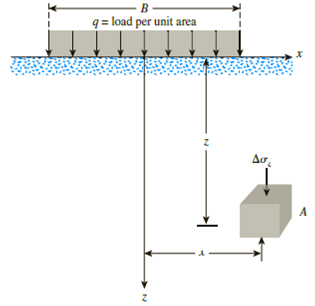Chapter 10, Problem 10.13PPrinciples of Geotechnical Enginee...

9th Edition
Braja M. Das + 1 other
ISBN: 9781305970939

Solutions

Chapter
SectionPrinciples of Geotechnical Enginee...

9th Edition
Braja M. Das + 1 other
ISBN: 9781305970939
Textbook Problem

Repeat Problem 10.12 for q = 700 kN/m2, B = 8 m, and z = 4 m. In this case, point A is located below the centerline under the strip load.10.12 Refer to Figure 10.43. A strip load of q = 1450 lb/ft2 is applied over a width with B = 48 ft. Determine the increase in vertical stress at point A located z = 21 ft below the surface. Given x = 28.8 ft.Figure 10.43

To determine

Calculate the increase in vertical stress at point A.

Explanation

Given information:

The strip load (q) is 700kN/m2.

The width of the load (B) is 8m.

The horizontal distance between centre of strip load q to point A (x) is 0.

The depth of the stress from ground surface (z) is 4m.

Calculation:

Calculate the ratio 2xB as shown below.

Substitute 0 for x and 8m for B.

2xB=2×08=0

Calculate the ratio 2zB as shown below.

Substitute 4m for z and 8m for B.

2zB=2×48=1

Calculate the increase in vertical stress (Δσz) using the relation as follows.

Refer Table 10.4 “Variation of Δσzq with 2zB and 2xB” in the Text Book

Still sussing out bartleby?

Check out a sample textbook solution.

See a sample solution

The Solution to Your Study Problems

Bartleby provides explanations to thousands of textbook problems written by our experts, many with advanced degrees!

Get Started

Find more solutions based on key concepts